Related Articles

# Subset or Filter data with multiple conditions in PySpark

• Last Updated : 16 May, 2021

Sometimes while dealing with a big dataframe that consists of multiple rows and columns we have to filter the dataframe, or we want the subset of the dataframe for applying operation according to our need. For getting subset or filter the data sometimes it is not sufficient with only a single condition many times we have to pass the multiple conditions to filter or getting the subset of that dataframe. So in this article, we are going to learn how ro subset or filter on the basis of multiple conditions in the PySpark dataframe.

To subset or filter the data from the dataframe we are using the filter() function. The filter function is used to filter the data from the dataframe on the basis of the given condition it should be single or multiple.

Syntax: df.filter(condition)

where df is the dataframe from which the data is subset or filtered.

We can pass the multiple conditions into the function in two ways:

• Using double quotes (“conditions”)
• Using dot notation in condition

Let’s create a dataframe.

## Python

 `# importing necessary libraries``from` `pyspark.sql ``import` `SparkSession`` ` `# function to create SparkSession``def` `create_session():``    ``spk ``=` `SparkSession.builder \``        ``.master(``"local"``) \``        ``.appName(``"Student_report.com"``) \``        ``.getOrCreate()``    ``return` `spk`` ` ` ` `def` `create_df(spark, data, schema):``    ``df1 ``=` `spark.createDataFrame(data, schema)``    ``return` `df1`` ` ` ` `if` `__name__ ``=``=` `"__main__"``:`` ` `    ``# calling function to create SparkSession``    ``spark ``=` `create_session()`` ` `    ``input_data ``=` `[(``1``, ``"Shivansh"``, ``"Male"``, ``20``, ``80``),``                  ``(``2``, ``"Arpita"``, ``"Female"``, ``18``, ``66``),``                  ``(``3``, ``"Raj"``, ``"Male"``, ``21``, ``90``),``                  ``(``4``, ``"Swati"``, ``"Female"``, ``19``, ``91``),``                  ``(``5``, ``"Arpit"``, ``"Male"``, ``20``, ``50``),``                  ``(``6``, ``"Swaroop"``, ``"Male"``, ``23``, ``65``),``                  ``(``7``, ``"Reshabh"``, ``"Male"``, ``19``, ``70``)]``    ``schema ``=` `[``"Id"``, ``"Name"``, ``"Gender"``, ``"Age"``, ``"Percentage"``]`` ` `    ``# calling function to create dataframe``    ``df ``=` `create_df(spark, input_data, schema)``    ``df.show()`

Output: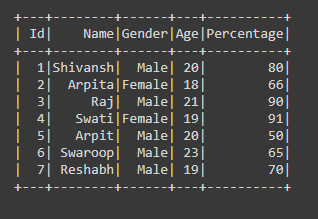Let’s apply the filter here:

Example 1: Using the and’ operator in (“”) double quotes

## Python

 `# subset or filter the dataframe by``# passing Multiple condition``df ``=` `df.``filter``(``"Gender == 'Male' and Percentage>70"``)``df.show()`

Output: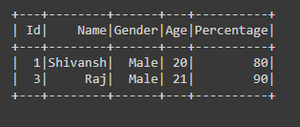Example 2: Using the ‘oroperator in (“”) double quotes

## Python

 `# subset or filter the data with``# multiple condition``df ``=` `df.``filter``(``"Age>20 or Percentage>80"``)``df.show()`

Output: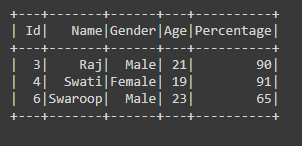Example 3: Using the ‘&operator with the (.) operator

## Python

 `# subset or filter the dataframe by``# passing Multiple condition``df ``=` `df.``filter``((df.Gender``=``=``'Female'``) & (df.Age>``=``18``))``df.show()`

Output: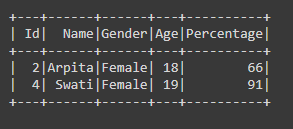Example 4: Using the ‘|operator with the (.) operator

## Python

 `# subset or filter the data with``# multiple condition``df ``=` `df.``filter``((df.Gender``=``=``'Male'``) | (df.Percentage>``90``))``df.show()`

Output: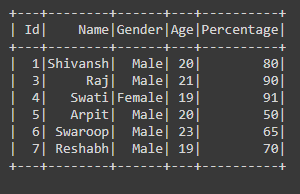Attention geek! Strengthen your foundations with the Python Programming Foundation Course and learn the basics.

To begin with, your interview preparations Enhance your Data Structures concepts with the Python DS Course. And to begin with your Machine Learning Journey, join the Machine Learning – Basic Level Course

My Personal Notes arrow_drop_up